# Engineering Graph PaperPaper Size: Letter

Format: PDF

Size: 8.5" x 11"  -  ( 216 mm x 279 mm )

Layout: Portrait / Vertical

Color: Gray

Engineering graph paper is a type of graph paper with horizontal and vertical lines at equal distance with major lines appearing at a specified distance of 1/2 inch, 1/4th, 1/5th, 1/10th, or every 5 or 10 lines.

This type of graph paper is perfect for all your mathematical and scientific needs. The grid on engineering graph paper is typically smaller than the grid on regular graph paper, while thick lines appear every few thin lines, which makes it easier to draw precise lines and curves.

## Why Use Engineering Graph Paper?

There are a few reasons why you might want to use engineering graph paper instead of regular graph paper.

• First, the smaller grid on engineering graph paper makes it easier to draw precise lines and curves.
• Second, the grid is typically calibrated in inches or millimeters, which makes it ideal for drafting engineering drawings and sketches.
• Finally, most versions of engineering graph paper feature a faint grid line that is easy to see but won't obscure your drawing.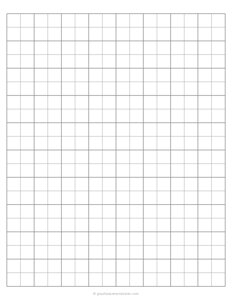## Half Inch (1/2")

Half Inch Engineering Graph Paper, with half inch squares and a major grid every two squares.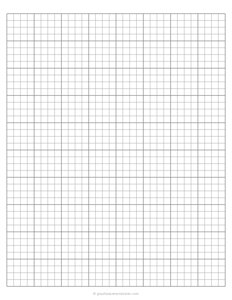## Quarter Inch (1/4")

Quarter Inch Engineering Graph Paper, with quarter inch squares and a major grid every four squares.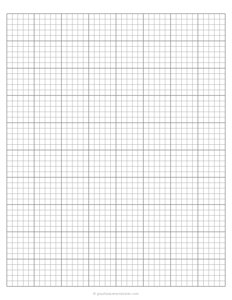## One Fifth (1/5")

1/5" Inch Engineering Graph Paper, with one fifth inch squares and a major grid every five squares.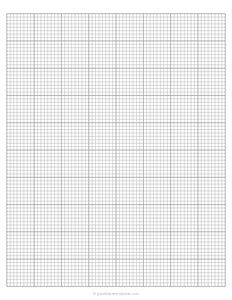## One Eighth (1/8")

1/8" Inch Graph Paper, with one eighth inch squares and a major grid every eight squares.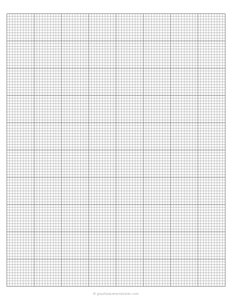## One Tenth (1/10")

1/10" Inch Graph Paper, with one tenth inch squares and a major grid every ten squares.

## How Do I Use Engineering Graph Paper?

The grid on engineering graph paper is typically divided into small squares, so you can use it to create accurate drawings and sketches. To use the paper, simply draw your design or diagram in the squares on the grid.

You can use a variety of drawing tools, such as a pencil, pen, or marker, to create your sketch. When you're finished, you can erase any mistakes or unwanted lines with an eraser or whiteout.

Engineering Graph Paper

### You may also like:

Narrow Ruled Lined Paper

Narrow Ruled Lined Paper, is a lined paper with blue horizontal lines with 6.4mm spacing and a vertical red line with a left and top margin of 32mm. Narrow spacing between horizontal lines makes it easy to fit more lines on one page.

1 cm Graph Paper

Free printable 1 cm graph paper (10mm spacing) with grey grid lines in portrait orientation. This type of graph paper has 1 cm squares, which makes it perfect for plotting out small-scale drawings and diagrams.

Semi-Log Graph Paper

Semi-log graph paper, also called semi-logarithmic or log linear graph paper, is a graphing paper with linear scales along the x-axis (horizontal axis) and logarithmic scales along the y-axis (vertical axis).

Quilt Grid Graph Paper (1" inch)

Quilt grid graph paper template with one line per inch is great for creating patterns and designs for your quilts. It is perfect for a beginner or seasoned quilter.

1/10" Inch Graph Paper PDF

Free printable 1/10 inch graph paper with grid lines in portrait orientation. This type of graph paper has 1/10 inch squares, which makes it perfect for math and science projects.